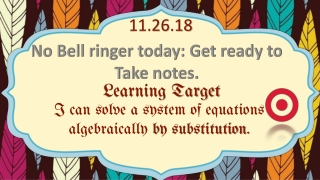DownloadDownload Presentation11.26.18

# 11.26.18

Download Presentation## 11.26.18

- - - - - - - - - - - - - - - - - - - - - - - - - - - E N D - - - - - - - - - - - - - - - - - - - - - - - - - - -
##### Presentation Transcript

1. 11.26.18 No Bell ringer today: Get ready to Take notes. Learning Target I can solve a system of equations algebraically by substitution.

2. AGENDA ~Systems by Substitution Notes ~Practice  ~IXL

3. Sometimes, graphing is not the best method to solve a system. What’s the sol?

4. Sometimes, graphing is not the best method to solve a system. What’s the sol?

5. When we need a precise measurement, or the graph isn’t a whole number, we can use the SUBSTITUTION method to solve systems.

6. Solving Systems of Equations by SUBSTITUTION Think “Substitute teacher” or subbing in a second string player for a hurt starter. One takes the place of the other.

7. Ex 1 When you Know x or y Value-halfway there!

8. Ex 1 When you Know x or y Value-halfway there!

9. Ex 2 y = -2 4x – 3y = 18

10. Ex 2 y = -2 4x – 3y = 18

11. What if the SUB is not just a number?

12. Ex3- When you Don’t know y’s value, But you CAN substitute 2x

13. Ex3- When you Don’t know y’s value, But you CAN substitute 2x

14. SUBSTITUTION Let’s say we have two equations, both equal to 5.

15. SUBSTITUTION Is it true that 7-2 is the same as 1(5)? We will be working from this simple example.

16. Solving Systems of Equations by SUBSTITUTION Since y equals “x-3” and y also Equals “2x” we can say x-3=2x, just like we did with 7-2=5(1)

17. Solving Systems of Equations by SUBSTITUTION Now solve for “x”

18. Solving Systems of Equations by SUBSTITUTION -x -x -3 = x Now solve for “x”

19. Solving Systems of Equations by SUBSTITUTION Since we know x, substitute into one of the equations to find “y” -x -x -3 = x

20. Solving Systems of Equations by SUBSTITUTION -x -x -3 = x Solution (-3,-6) y = -6

21. CHECKING: Solution (-3,-6) -6 true true

22. Solution (-3,-6) -6 true true We just used SUBSTITUTION to solve the system. This example is also the TRANSITIVE PROPERTY.

23. Ex 4

24. Ex 4

25. Practice 2. 1. 2

26. Reflection on Padlet Post a picture of a real-world situation that requires a substitute be unique-if it is already posted, or given in class, it isn't an option. Then, IXL AA.8 to an 85% https://padlet.com/poolec/subs2nd

27. GEOME-TREE!  Review for Semester Test. Choose from the following categories: EQUATIONS SLOPE-INTERCEPT PYTHAGOREAN THEOREM RATIONAL/IRRATIONAL